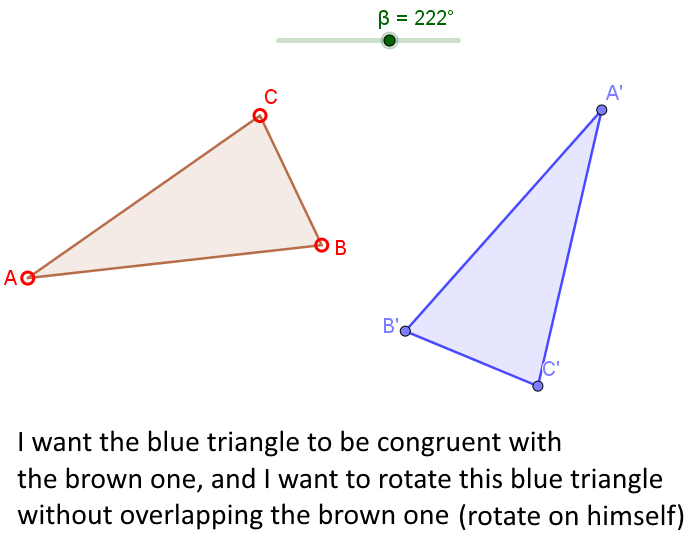# How to make two triangles/polygon same?

Math123 shared this question 9 months ago

How to make two triangles (points , angle and lengths) same with some distance also i can rotate 2nd triangle and they do not overlap. If i change position , length and angle of one triangle it automatically change 2nd and keep triangles congruent.

2nd triangle should have some rotation (can adjust rotation) and distance with respect to 1st triangle so they do not overlap.1

Example here : https://www.geogebra.org/m/qn7zxva8

Vector MM' allows you to change distance between first and second triangle.

If you want to see the vertices of the 2nd and 3rd triangles, change the definition of triangle1 from Polygon({A,B,C}) to Polygon(A,B,C).

I spent 10 min. to make this example (so it's more or less OK). But I don't know if it's what you need ...

So, generally, send a file and be very precise in your request.1

Pls send a sample for the first triangle.

Pls describe the rules for the Displacement between the 2 triangles (example given manually with Point, given with direction and automatic distance etc.)1

Could be any triangle but 2nd triangle is same in angle and length (congruent). Any distance (only because both triangles do not overlap). and 2nd triangle can be rotated (to demonstrate rotation does not effect congruence). 2nd triangle also have points to show correspondence.1

Did you have a look at my example ? Is it OK for you or not ?

Please post a file so that we can clearly see what your problem is.1

Thanks both Pierre Rubod and rami for the support. I found this link solved my problem.

https://www.geogebra.org/m/...1

a possiblity more1

Finding a solution is always a good news. :smile:

rami and me did not understand your problem in the same way, and rami's solution is the good one for you.

So, in this example, you can see that it is important to first search in the GGB data base, and if you find nothing, you have to be very precise in your request, even in posting a drawing or a simple GGB file describing your problem. :wink:

For example :

(picture from rami's file)Regards,

Pierre1

try with attached1

Thanks Peirre and alfabeta. Your solutions worked for me and i learned a lot.

and Specially @rami you are always awesome. Give your software expertise to me. So i can easily picturize anything with GGB whatever my ideas or in my mind.